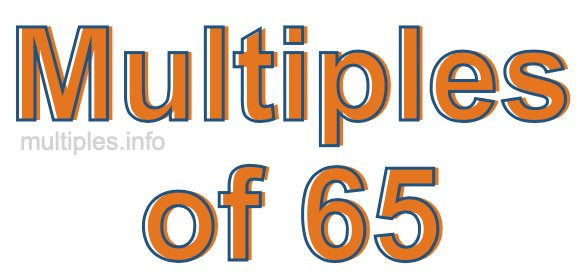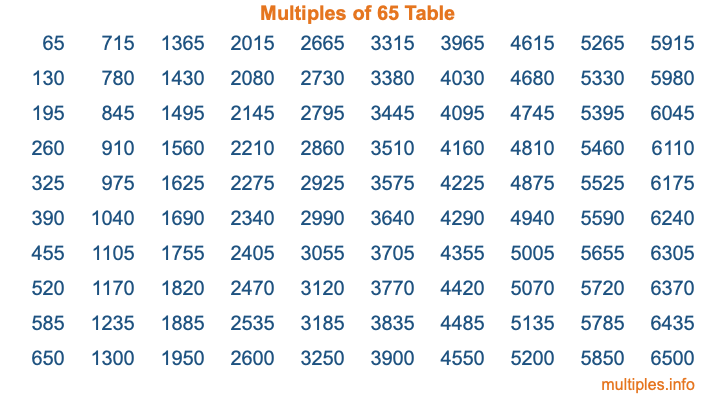Multiples of 65Welcome to the Multiples of 65 page. Here we will first teach you everything you will ever need to know about the multiples of 65, and then give you a study guide summary of everything we taught you to make sure you remember it all. Use this page to look up facts and learn information about the multiples of 65. This page will make you a multiples of sixty-five expert!

Definition of Multiples of 65
Multiples of 65 are all the numbers that when divided by 65 equal an integer. Each of the multiples of 65 are called a multiple. A multiple of 65 is created by multiplying 65 by an integer.

Therefore, to create a list of multiples of 65, you start with 1 multiplied by 65, then 2 multiplied by 65, then 3 multiplied by 65, and so on for as long as you want. Thus, the list of the first five multiples of 65 is 65, 130, 195, 260, and 325. To see a larger list of multiples of 65, see the printable image of Multiples of 65 further down on this page. We also have a category where you can choose any nth multiple of 65.

Multiples of 65 Checker
The Multiples of 65 Checker below checks to see if any number of your choice is a multiple of 65. In other words, it checks to see if there is any number (integer) that when multiplied by 65 will equal your number. To do that, we divide your number by 65. If the the quotient is an integer, then your number is a multiple of 65.

Is  a multiple of 65?

Least Common Multiple of 65 and ...
A Least Common Multiple (LCM) is the lowest multiple that two or more numbers have in common. This is also called the smallest common multiple or lowest common multiple and is useful to know when you are adding our subtracting fractions. Enter one or more numbers below (65 is already entered) to find the LCM.

Check out our LCM Calculator if you need more details about the Least Common Multiple or if you need the LCM for different numbers for adding and subtraction fractions.

nth Multiple of 65
As we stated above, 65 is the first multiple of 65, 130 is the second multiple of 65, 195 is the third multiple of 65, and so on. Enter a number below to find the nth multiple of 65.

th multiple of 65

Multiples of 65 vs Factors of 65
65 is a multiple of 65 and a factor of 65, but that is where the similarities end. All postive multiples of 65 are 65 or greater than 65. All positive factors of 65 are 65 or less than 65.

Below is the beginning list of multiples of 65 and the factors of 65 so you can compare:

Multiples of 65: 65, 130, 195, 260, 325, etc.

Factors of 65: 1, 5, 13, 65

As you can see, the multiples of 65 are all the numbers that you can divide by 65 to get a whole number. The factors of 65, on the other hand, are all the whole numbers that you can multiply by another whole number to get 65.

It's also interesting to note that if a number (x) is a factor of 65, then 65 will also be a multiple of that number (x).

Multiples of 65 vs Divisors of 65
The divisors of 65 are all the integers that 65 can be divided by evenly. Below is a list of the divisors of 65.

Divisors of 65: 1, 5, 13, 65

The interesting thing to note here is that if you take any multiple of 65 and divide it by a divisor of 65, you will see that the quotient is an integer.

Multiples of 65 Table
Below is an image of the first 100 multiples of 65 in a table. The table is in chronological order, column by column. The first column has the first ten multiples of 65, the second column has the next ten multiples of 65, and so on.The Multiples of 65 Table is also referred to as the 65 Times Table or Times Table of 65. You are welcome to print out our table for your studies.

Negative Multiples of 65
Although not often discussed or needed in math, it is worth mentioning that you can make a list of negative multiples of 65 by multiplying 65 by -1, then by -2, then by -3, and so on, to get the following list of negative multiples of 65:

-65, -130, -195, -260, -325, etc.

Multiples of 65 Summary
Below is a summary of important Multiples of 65 facts that we have discussed on this page. To retain the knowledge on this page, we recommend that you read through the summary and explain to yourself or a study partner why they hold true.

There are an infinite number of multiples of 65.

A multiple of 65 divided by 65 will equal a whole number.

65 divided by a factor of 65 equals a divisor of 65.

The nth multiple of 65 is n times 65.

The largest factor of 65 is equal to the first positive multiple of 65.

65 is a multiple of every factor of 65.

65 is a multiple of 65.

A multiple of 65 divided by a divisor of 65 equals an integer.

65 divided by a divisor of 65 equals a factor of 65.

Any integer times 65 will equal a multiple of 65.

Multiples of a Number
Here you can get the multiples of another number, all with the same attention to detail as we did for multiples of 65 on this page.

Multiples of
Multiples of 66
Did you find our page about multiples of sixty-five educational? Do you want more knowledge? Check out the multiples of the next number on our list!